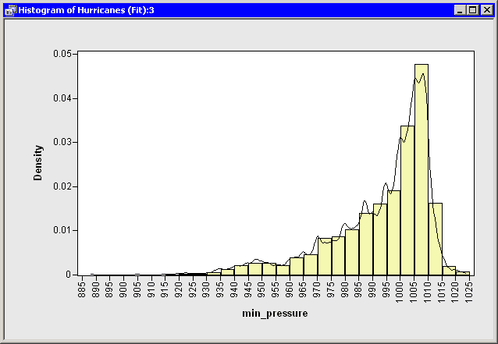## Draw a Curve from Variables in a Data Set or from a SAS/IML Matrix

Sometimes the number of observations in your data is different from the number of observations in an output data set created by a procedure. This eliminates the possibility of reading the output into the DataObject that contains the original data.

For example, you can use the SCORE procedure to evaluate a linear model on a separate set of values of the explanatory variables. Or you can use the SCORE statement of the LOESS, GAM, or TPSPLINE procedure to evaluate a nonparametric model. In each of these cases, you can read variables from the output data set by using the SAS/IML USE and READ statements and then use the DrawLine method to add the relevant curve to a plot.

As an example of this approach, suppose you want to add a kernel density estimate to a histogram of the `min_pressure` variable. You can do this by calling the KDE procedure. Add the following statements at the bottom of the program window, and select ProgramRun from the main menu.

```
submit;
proc kde data=Hurr;
univar min_pressure / out=KDEOut plots=none;
run;
endsubmit;
```

The KDE procedure creates the data set `Work.KDEout` on the SAS server with 401 observations. The data set contains a variable `Value` that consists of evenly spaced points between the minimum and maximum values of `min_pressure`. A variable named `Density` contains the kernel density estimate evaluated at the points of `Value`.

Note: You do not need to use the SORT procedure to sort this data set by `Value`, because it was created in sorted order. However, for unsorted data, you need to sort by the independent variable.

A straightforward approach is to create a histogram and add the kernel density estimate by reading in the `Work.KDEout` data set. Add the following statements at the bottom of the program window, and select ProgramRun from the main menu.

```
use KDEOut;
close KDEOut;

declare Histogram hist;
hist = Histogram.Create( dobj, "min_pressure" );
hist.ShowDensity(); /* show density instead of frequency */
hist.DrawUseDataCoordinates();
hist.DrawLine( value, density );
```

Figure 6.4 displays the resulting histogram and the overlaid kernel density estimate.

Figure 6.4: A Histogram Overlaid with a Kernel Density Estimate